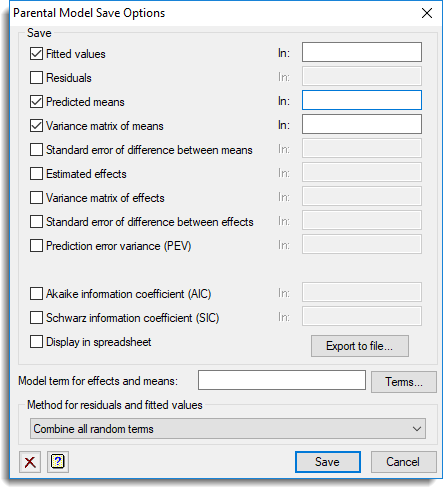1. Home
2. Parental Model Save Options

# Parental Model Save Options

Use this to save results from a fitting a parental model in Genstat data structures. After selecting the appropriate boxes, you need to type the identifiers of the data structures into the corresponding In: fields.## Save

 Fitted values Variate Fitted values from the analysis Residuals Variate Residuals from the analysis Predicted means Table Predicted means for the specified term Variance matrix of means Symmetric matrix Variance-covariance matrix for the predicted means Standard error of difference between means Symmetric matrix Standard errors of differences between the predicted means Estimated effects Table Estimated regression coefficients for the specified term Variance matrix of effects Symmetric matrix Variance-covariance matrix for the estimated effects Standard error of difference between effects Symmetric matrix Standard errors of differences between the estimated parameters of each term Predicted error variance Diagonal matrix Predicted error variance for the specified term Akaike information coefficient (AIC) Scalar Akaike information coefficient to assess the random model Schwarz information coefficient (SIC) Scalar Schwarz information coefficient to assess the random model

When selected the data structures that are saved will be displayed within a spreadsheet.

## Model terms for effects and means

Specifies the model term for which tables of estimated effects and predicted means are to be saved. The string ‘Constant’ may be used to save results for the constant term. If tables of effects or means are required for more than one model term, this menu should be invoked once for each term, changing the specification of the model term each time.

## Form residuals

Residuals can be formed from either all random terms, or just the final residual stratum (default).

Updated on January 26, 2022